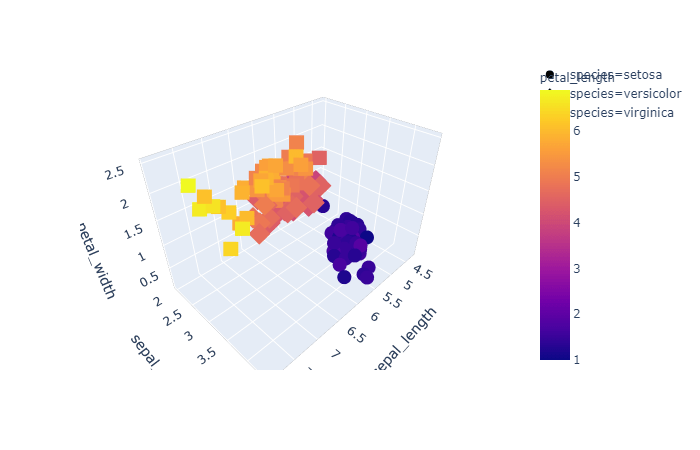# How to Create 3d Interactive Graph using Plotly

Like matplotlib and seaborn we have plotly, that is a data visualization library used for creating graphical, 3d and interactive graph.

You can install plotly using below command

``pip install plotly``

Then you can import it in Jupyter notebook as below.

``import plotly.express as pex``

Let us use the famous iris dataset to create the 3d and interactive graph

``iris_data_set = pex.data.iris()``

Finally we need to generate the graph using below code.

``````my_plot = pex.scatter_3d(iris_data_set, x='sepal_length',y='sepal_width',z='petal_width',color='petal_length',symbol='species')
my_plot.show()``````